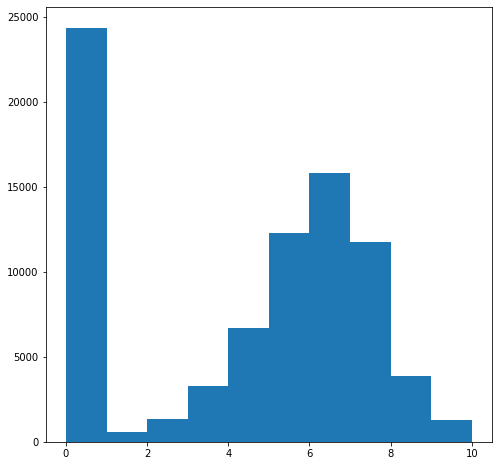## Required Packages

import sys
import numpy as np
import matplotlib as mpl
import matplotlib.pyplot as plt
import seaborn as sns
import pandas as pd
import sklearn

plt.rcParams['figure.figsize'] = (8, 8)

from sklearn.model_selection import train_test_split


## Version Check

print('Python: {}'.format(sys.version))
print('Numpy: {}'.format(np.__version__))
print('Matplotlib: {}'.format(mpl.__version__))
print('Seaborn: {}'.format(sns.__version__))
print('Pandas: {}'.format(pd.__version__))
print('Scikit-learn: {}'.format(sklearn.__version__))

Python: 3.7.6 (default, Jan  8 2020, 20:23:39) [MSC v.1916 64 bit (AMD64)]
Numpy: 1.18.1
Matplotlib: 3.1.3
Seaborn: 0.10.0
Pandas: 1.0.1
Scikit-learn: 0.22.1


More data information is in here

games = pd.read_csv('./dataset/games.csv')

id type name yearpublished minplayers maxplayers playingtime minplaytime maxplaytime minage users_rated average_rating bayes_average_rating total_owners total_traders total_wanters total_wishers total_comments total_weights average_weight
0 12333 boardgame Twilight Struggle 2005.0 2.0 2.0 180.0 180.0 180.0 13.0 20113 8.33774 8.22186 26647 372 1219 5865 5347 2562 3.4785
1 120677 boardgame Terra Mystica 2012.0 2.0 5.0 150.0 60.0 150.0 12.0 14383 8.28798 8.14232 16519 132 1586 6277 2526 1423 3.8939
2 102794 boardgame Caverna: The Cave Farmers 2013.0 1.0 7.0 210.0 30.0 210.0 12.0 9262 8.28994 8.06886 12230 99 1476 5600 1700 777 3.7761
3 25613 boardgame Through the Ages: A Story of Civilization 2006.0 2.0 4.0 240.0 240.0 240.0 12.0 13294 8.20407 8.05804 14343 362 1084 5075 3378 1642 4.1590
4 3076 boardgame Puerto Rico 2002.0 2.0 5.0 150.0 90.0 150.0 12.0 39883 8.14261 8.04524 44362 795 861 5414 9173 5213 3.2943

## Exploratory Data Analysis

games.describe()

id yearpublished minplayers maxplayers playingtime minplaytime maxplaytime minage users_rated average_rating bayes_average_rating total_owners total_traders total_wanters total_wishers total_comments total_weights average_weight
count 81312.000000 81309.000000 81309.000000 81309.000000 81309.000000 81309.000000 81309.000000 81309.000000 81312.000000 81312.000000 81312.000000 81312.000000 81312.000000 81312.000000 81312.000000 81312.000000 81312.000000 81312.000000
mean 72278.150138 1806.630668 1.992018 5.637703 51.634788 49.276833 51.634788 6.983975 161.886585 4.212144 1.157632 262.502509 9.236423 12.688890 42.719144 49.290031 16.488009 0.908083
std 58818.237742 588.517834 0.931034 56.076890 345.699969 334.483934 345.699969 5.035138 1145.978126 3.056551 2.340033 1504.536693 39.757408 60.764207 239.292628 284.862853 115.980285 1.176002
min 1.000000 -3500.000000 0.000000 0.000000 0.000000 0.000000 0.000000 0.000000 0.000000 0.000000 0.000000 0.000000 0.000000 0.000000 0.000000 0.000000 0.000000 0.000000
25% 21339.750000 1984.000000 2.000000 2.000000 8.000000 10.000000 8.000000 0.000000 0.000000 0.000000 0.000000 1.000000 0.000000 0.000000 0.000000 0.000000 0.000000 0.000000
50% 43258.000000 2003.000000 2.000000 4.000000 30.000000 30.000000 30.000000 8.000000 2.000000 5.265620 0.000000 7.000000 0.000000 0.000000 1.000000 1.000000 0.000000 0.000000
75% 128836.500000 2010.000000 2.000000 6.000000 60.000000 60.000000 60.000000 12.000000 16.000000 6.718777 0.000000 51.000000 2.000000 3.000000 7.000000 9.000000 2.000000 1.916700
max 184451.000000 2018.000000 99.000000 11299.000000 60120.000000 60120.000000 60120.000000 120.000000 53680.000000 10.000000 8.221860 73188.000000 1395.000000 1586.000000 6402.000000 11798.000000 5996.000000 5.000000
print(games.columns)
print(games.shape)

Index(['id', 'type', 'name', 'yearpublished', 'minplayers', 'maxplayers',
'playingtime', 'minplaytime', 'maxplaytime', 'minage', 'users_rated',
'average_rating', 'bayes_average_rating', 'total_owners',
'total_weights', 'average_weight'],
dtype='object')
(81312, 20)


Our purpose is to predict average_rating. But some rows contain 0 rating. So we should remove that.

plt.hist(games['average_rating']);print(games[games['average_rating'] == 0].iloc)

id                             318
type                     boardgame
name                    Looney Leo
yearpublished                    0
minplayers                       0
maxplayers                       0
playingtime                      0
minplaytime                      0
maxplaytime                      0
minage                           0
users_rated                      0
average_rating                   0
bayes_average_rating             0
total_owners                     0
total_wanters                    0
total_wishers                    1
total_weights                    0
average_weight                   0
Name: 13048, dtype: object


This row is meaningless

print(games[games['average_rating'] > 0].iloc)

id                                  12333
type                            boardgame
name                    Twilight Struggle
yearpublished                        2005
minplayers                              2
maxplayers                              2
playingtime                           180
minplaytime                           180
maxplaytime                           180
minage                                 13
users_rated                         20113
average_rating                    8.33774
bayes_average_rating              8.22186
total_owners                        26647
total_wanters                        1219
total_wishers                        5865
total_weights                        2562
average_weight                     3.4785
Name: 0, dtype: object

games = games[games['users_rated'] > 0]

# Remove any rows with missing values
games.dropna(axis=0, inplace=True)

# Make a histogram of all the average ratings
plt.hist(games['average_rating']);corrmat = games.corr()

fig = plt.figure(figsize=(12, 9))
sns.heatmap(corrmat, vmax=.8, square=True);## Preprocess Dataset

columns = games.columns.tolist()

# Filter the columns to remove data we don't want
columns = [c for c in columns if c not in ['bayes_average_rating', 'average_rating', 'type', 'name', 'id']]

# Store the variable we'll be predicting on
target = 'average_rating'

train = games.sample(frac=0.8, random_state=1)
test = games.loc[~games.index.isin(train.index)]

# Print shapes
print(train.shape)
print(test.shape)

(45515, 20)
(11379, 20)


## Build Linear Regression Model

from sklearn.linear_model import LinearRegression
from sklearn.metrics import mean_squared_error

# Initialize the model class
lr = LinearRegression()

# Fit the model with training data
lr.fit(train[columns], train[target])

LinearRegression(copy_X=True, fit_intercept=True, n_jobs=None, normalize=False)
predictions = lr.predict(test[columns])

# Compute error between test predictions and actual values
print('MSE : {}'.format(mean_squared_error(predictions, test[target])))

MSE : 2.0788190326293257


## Build Non-Linear Regression (RandomForestRegressor) Model

from sklearn.ensemble import RandomForestRegressor

# Initialize the model class
rfr = RandomForestRegressor(n_estimators=100, min_samples_leaf=10, random_state=1)

# Fit the model with training data
rfr.fit(train[columns], train[target])

RandomForestRegressor(bootstrap=True, ccp_alpha=0.0, criterion='mse',
max_depth=None, max_features='auto', max_leaf_nodes=None,
max_samples=None, min_impurity_decrease=0.0,
min_impurity_split=None, min_samples_leaf=10,
min_samples_split=2, min_weight_fraction_leaf=0.0,
n_estimators=100, n_jobs=None, oob_score=False,
random_state=1, verbose=0, warm_start=False)
predictions = rfr.predict(test[columns])

# Compute error between test predictions and actual values
print('MSE : {}'.format(mean_squared_error(predictions, test[target])))

MSE : 1.4458560046071653


As a result, we can get more improved result from non-linear regression rather than linear regression model.

## Validate the model with individual test set

test[columns].iloc

yearpublished      2008.0000
minplayers            1.0000
maxplayers            5.0000
playingtime         200.0000
minplaytime         100.0000
maxplaytime         200.0000
minage               12.0000
users_rated       15774.0000
total_owners      16429.0000
total_wanters      1343.0000
total_wishers      5149.0000
total_weights      1450.0000
average_weight        3.7531
Name: 14, dtype: float64
rating_lr = lr.predict(test[columns].iloc.values.reshape(1, -1))
rating_rfr = rfr.predict(test[columns].iloc.values.reshape(1, -1))

# Print out the predictions
print(rating_lr)
print(rating_rfr)

[9.20860328]
[7.85532168]

print(test[target].iloc)

7.99115